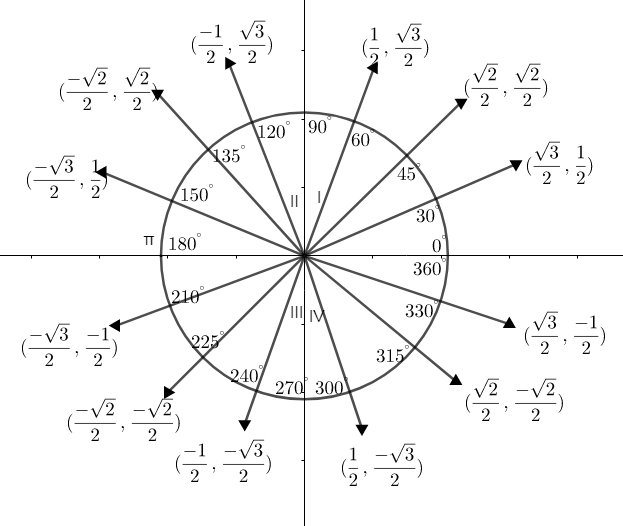Courses
Courses for Kids
Free study material
Free LIVE classes
MoreLIVE
Join Vedantu’s FREE Mastercalss

# Find the value of$\sin {{120}^{\circ }}$.A) $\dfrac{\sqrt{3}}{2}$B) $-\dfrac{\sqrt{3}}{2}$C) $\dfrac{1}{2}$D) $-\dfrac{1}{2}$Verified
361.2k+ views
Hint: Draw a unit circle and chart out the trigonometric values on each quadrant of the circle. Find the value of $\sin {{120}^{\circ }}$ or the value of $\sin {{120}^{\circ }}$can be taken from the trigonometric table. Find either sine function or cosine function.

By using a unit circle we can find the value of $\sin {{120}^{\circ }}$. Now let us draw a cartesian plane with $x=\cos \theta$ and $y=\sin \theta$.
Let us draw the trigonometric table as well:

 sin cos tan cot sec Cosec 0 0 1 0 N.A 1 N.A 30 $\dfrac{1}{2}$ $\dfrac{\sqrt{3}}{2}$ $\dfrac{1}{\sqrt{3}}$ $\sqrt{3}$ $\dfrac{2\sqrt{3}}{3}$ 2 45 $\dfrac{1}{\sqrt{2}}$ $\dfrac{1}{\sqrt{2}}$ 1 1 $\sqrt{2}$ $\sqrt{2}$ 60 $\dfrac{\sqrt{3}}{2}$ $\dfrac{1}{2}$ $\sqrt{3}$ $\dfrac{\sqrt{3}}{3}$ 2 $\dfrac{2\sqrt{3}}{3}$ 90 1 0 N.A 0 N.A 1

Now let us mark their values in the unit circle.Here, $x=\cos \theta ,y=\sin \theta$.
Eg: -$\left( \cos {{30}^{\circ }},\sin {{30}^{\circ }} \right)=\left( x,y \right)$
$\left( x,y \right)=\left( \dfrac{\sqrt{3}}{2},\dfrac{1}{2} \right)$.
In the first quadrant, the values of $\cos \theta$ and $\sin \theta$ are positive.
In the second quadrant, the value of $\cos \theta$ is negative and $\sin \theta$ is positive.
In the third quadrant, both are negative.
In the fourth quadrant, $\cos \theta$ is positive and $\sin \theta$ is negative.
By looking into the figure, you can find that $\sin 60=\sin 120$.
i.e. $\sin 60=\sin 120=\dfrac{\sqrt{3}}{2}$
Or if we are directly taking value from the trigonometric table, we need to find the value of $\sin {{120}^{\circ }}$ by using other angles of sin functions such as ${{60}^{\circ }}$ and $\sin {{180}^{\circ }}$.
We know that ${{180}^{\circ }}-{{60}^{\circ }}={{120}^{\circ }}$.
We also know that the trigonometric identity:
$\sin \left( 180-\theta \right)=\sin \theta$.
Put, $\theta ={{120}^{\circ }}$.
\begin{align} & \Rightarrow \sin \left( 180-120 \right)=\sin {{120}^{\circ }} \\ & \Rightarrow \sin {{60}^{\circ }}=\sin {{120}^{\circ }}=\dfrac{\sqrt{3}}{2} \\ \end{align}
From the trigonometric table, find the value of $\sin {{60}^{\circ }}=\dfrac{\sqrt{3}}{2}$.
$\therefore$Value of $\sin {{120}^{\circ }}=\dfrac{\sqrt{3}}{2}$
$\therefore$Option (a) is the correct answer.
Note:
We can also find the value of $\sin {{120}^{\circ }}$ by using cosine function.
Using the trigonometry formula,
$\sin \left( 90+\theta \right)=\cos \theta$
Thus to find the values of $\sin {{120}^{\circ }}$, put $\theta ={{30}^{\circ }}$
as, ${{90}^{\circ }}+{{30}^{\circ }}={{120}^{\circ }}$
\begin{align} & \Rightarrow \sin \left( 90+30 \right)=\cos 30 \\ & \sin {{120}^{\circ }}=\cos {{30}^{\circ }} \\ \end{align}
From trigonometric table, value of $\cos 30=\dfrac{\sqrt{3}}{2}$
$\therefore \sin {{120}^{\circ }}=\dfrac{\sqrt{3}}{2}$.
Last updated date: 25th Sep 2023
Total views: 361.2k
Views today: 3.61k